﻿ 多悬臂梁–流体的模态耦合与拍振研究 Research on Modal Coupling and Slap Phenomenon of Multi-Cantilever Beam-Fluid Structure

International Journal of Mechanics Research
Vol. 08  No. 03 ( 2019 ), Article ID: 32030 , 8 pages
10.12677/IJM.2019.83020

Research on Modal Coupling and Slap Phenomenon of Multi-Cantilever Beam-Fluid Structure

Yi Lu, Nansheng Li, Yuchun Li*

Department of Hydraulic Engineering, School of Civil Engineering, Tongji University, Shanghai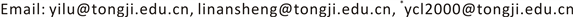Received: Aug. 14th, 2019; accepted: Aug. 28th, 2019; published: Sep. 4th, 2019ABSTRACT

In this paper, the modal analysis of multi-cantilever beam and fluid system is carried out by ANSYS code. The variations of wet frequencies of coupling system with the fluid width are obtained. The results agree well with the theoretical solutions. The beat vibration of multi-cantilever and fluid system is studied. In multi-cantilever beam and fluid system, not only there is interaction between beam and fluid, but also the interaction between the beams. The interaction effect between beams decreases with the increase of fluid width between beams. The difference of modal frequency caused by the interaction between beams results in beat vibration of multi-cantilever and fluid system. Because the different contributions of mode shapes are given to the displacement functions of different beams, the frequency and amplitude of beat vibration are generally different for the different beams.

Keywords:Multi-Cantilever Beam, Fluid, Modal Coupling, Beat Vibration1. 引言

2. 多悬臂梁–流体的模态耦合

2.1. 双悬悬臂梁–流体的模态耦合Table 1. The first two frequencies of cantilever beam with one side infinite waterFigure 1. Double cantilever beam-fluid system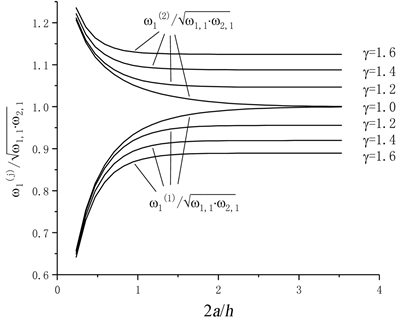Figure 2. Frequency variation curve of two modes of the first order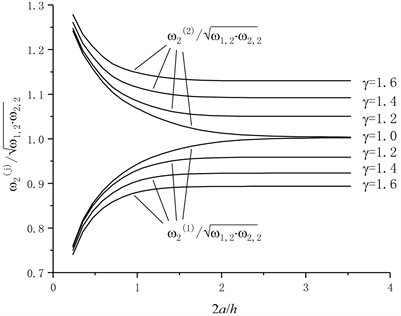Figure 3. Frequency variation curve of two modes of the second order

ANSYS模拟得到的曲线与文献  中理论解法得到的结果一致，结果表明：两个梁组成的一个系统，其系统某一阶自然频率有两个，当梁间距较小时，由于液体的耦合作用，系统的两个频率发生了很大变化，说明两个梁通过液体耦合发生了相互作用；随着梁间距增大，2a/h大于3时，系统的两个频率趋于直线，与单个梁与无穷水体作用的自然频率相同，说明梁间的耦合作用趋于零。

2.2. 三悬臂梁–流体结构模态影响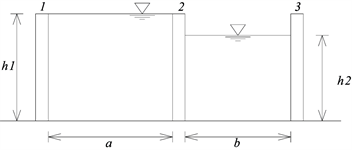Figure 4. Triple cantilever beam-fluid systemFigure 5. The three vibration shapes of the first mode shape for the system of triple cantilever beam and fluid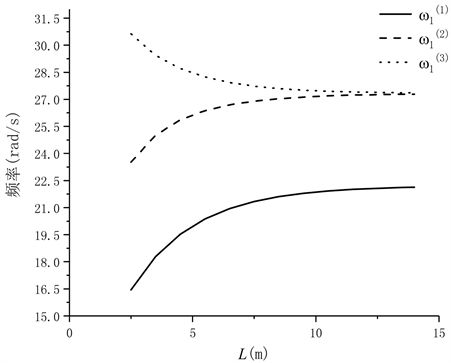Figure 6. Variations of the three wet frequencies with liquid width L

3. 耦合自由振动的拍现象

3.1. 双梁–液体系统拍振现象

$\left\{\begin{array}{l}{u}_{a}\left(z,t\right)=\underset{i=1}{\overset{\infty }{\sum }}{q}_{i}{Y}_{ai}\left(z\right)\cdot \mathrm{cos}\left({\omega }_{i}\cdot t+{\phi }_{i}\right)\\ {u}_{b}\left(z,t\right)=\underset{i=1}{\overset{\infty }{\sum }}{q}_{i}{Y}_{bi}\left(z\right)\cdot \mathrm{cos}\left({\omega }_{i}\cdot t+{\phi }_{i}\right)\end{array}$ (1)

$\left\{\begin{array}{l}{u}_{a}\left(z,t\right)={q}_{1}{Y}_{a1}\left(z\right)\cdot \mathrm{cos}\left({\omega }_{1}^{\left(1\right)}\cdot t+{\phi }_{1}\right)+{q}_{2}{Y}_{a2}\left(z\right)\cdot \mathrm{cos}\left({\omega }_{1}^{\left(2\right)}\cdot t+{\phi }_{2}\right)\\ {u}_{b}\left(z,t\right)={q}_{1}{Y}_{b1}\left(z\right)\cdot \mathrm{cos}\left({\omega }_{1}^{\left(1\right)}\cdot t+{\phi }_{1}\right)+{q}_{2}{Y}_{b2}\left(z\right)\cdot \mathrm{cos}\left({\omega }_{1}^{\left(2\right)}\cdot t+{\phi }_{2}\right)\end{array}$ (2)

${u}_{a}\left(z,0\right)=Y\left(z\right)$ ; ${\frac{\text{d}{u}_{a}}{\text{d}t}|}_{t=0}={{u}_{b}|}_{t=0}={\frac{\text{d}{u}_{b}}{\text{d}t}|}_{t=0}=0$ (3)

$\left\{\begin{array}{l}{u}_{a}=Y\left(z\right)\mathrm{cos}\left(\frac{{\omega }_{1}^{\left(2\right)}-{\omega }_{1}^{\left(1\right)}}{2}\cdot t\right)\mathrm{cos}\left(\frac{{\omega }_{1}^{\left(2\right)}+{\omega }_{1}^{\left(1\right)}}{2}\cdot t\right)\\ {u}_{b}=-Y\left(z\right)\mathrm{sin}\left(\frac{{\omega }_{1}^{\left(2\right)}-{\omega }_{1}^{\left(1\right)}}{2}\cdot t\right)\mathrm{sin}\left(\frac{{\omega }_{1}^{\left(2\right)}+{\omega }_{1}^{\left(1\right)}}{2}\cdot t\right)\end{array}$ (4)

3.2. 三梁–液体系统拍振现象

4. 结论

1) 由于流固耦合作用的存在，悬臂梁之间存在相互作用，这种相互作用影响了耦联结构的湿模态，且这种影响随着梁间液体宽度的增加而减弱。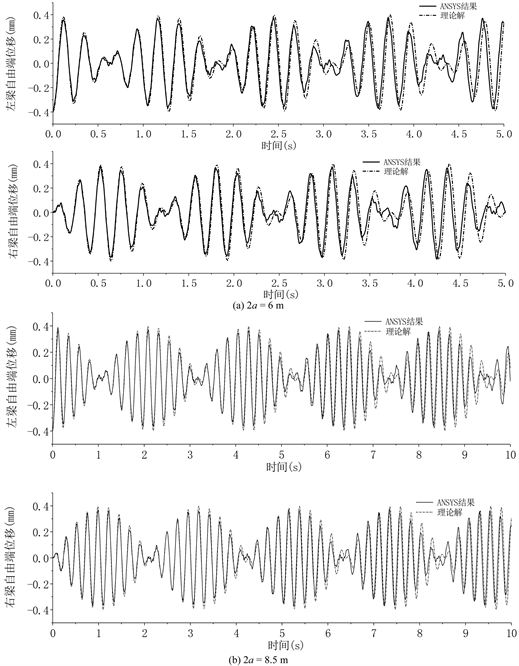Figure 7. Beat vibration of double beam-fluid system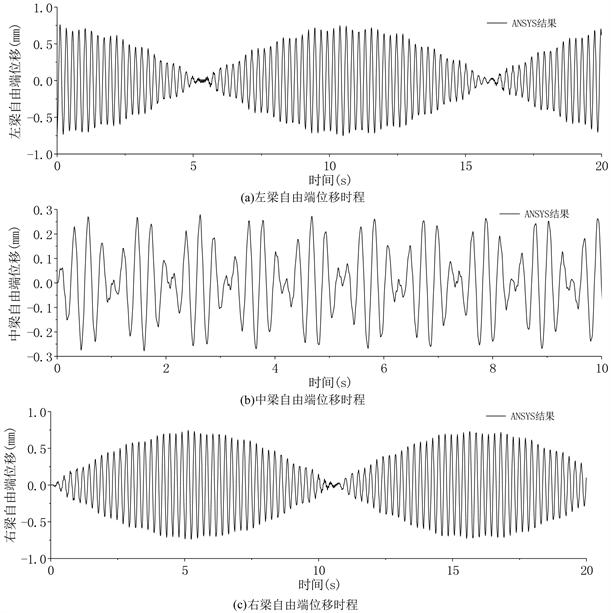Figure 8. Beat vibration of triple beam-fluid system

2) 多悬臂梁–流体耦联系统拍振现象是由梁间相互作用产生的模态频率差导致，由于不同模态对不同梁位移函数贡献不同，不同梁的拍现象频率及振幅一般不同。

Research on Modal Coupling and Slap Phenomenon of Multi-Cantilever Beam-Fluid Structure[J]. 力学研究, 2019, 08(03): 179-186. https://doi.org/10.12677/IJM.2019.83020

1. 1. 郑哲敏, 马宗魁. 悬臂梁在一侧受有液体作用时的自由振动[J]. 力学学报, 1959, 3(2): 111-119.

2. 2. 周叮. 两侧受液时悬臂梁的自由振动分析[J]. 工程力学, 1981, 8(3): 107-115.

3. 3. 李遇春, 朱暾, 白冰. 悬臂梁–流体–悬臂梁耦联系统的湿模态相互作用[J]. 工程力学, 1998, 15(1): 58-65.

4. 4. Meirovitch, L. (1975) Elements of Vibra-tion Analysis. Second Edition, McGraw-Hill Book Company, New York, 125-129.

5. 5. 李京颍. 拍现象的数值模拟分析[J]. 阜阳师范学院学报(自然科学版), 2005, 22(3): 17-19.

6. 6. 李遇春. 液体晃动动力学基础[M]. 北京: 科学出版社, 2017: 121-126.

7. 7. 王新敏. ANSYS工程结构数值分析[M]. 北京: 人民交通出版社, 2007: 273-278.

8. NOTES

*通讯作者。